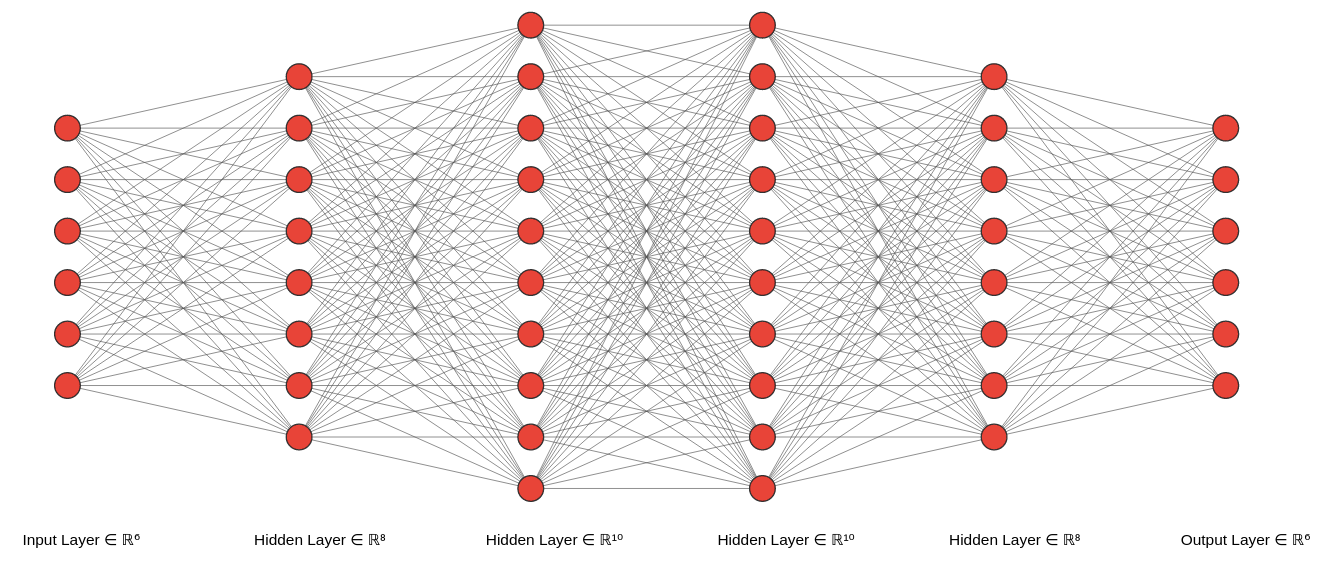This tutorial is a step-by-step guide to create, train and evaluate a CNN Model with TensorFlow. Mainly there are 3 approaches to define a convolutional neural network with TensorFlow.

The best way to become comfortable to define a CNN at the end of this post is to try each step yourself while going through each step and The recommended way is to use a Google Colab notebook so you won’t need any installation on your pc.

## Step 1 – Prepare Training and Test Dataset

For this tutorial, we’ll use CIFAR10 consists of natural images with 10 different classes. This dataset has 50,000 Training Images and 10,000 Test Images. and each image is 32 by 32 (Width x Height) pixels and has 3 channels so a colored Image.

First, Load TensorFlow and CIFAR10 dataset library

``````import tensorflow as tf
from tensorflow.keras.datasets import cifar10``````

Use load_data() function to retrieve the training and test images.

``(x_train, y_train), (x_test, y_test) = cifar10.load_data()``

Check the size of training images x_train and classes labels y_train.

``````print(x_train.shape)
print(y_train.shape)
# Output
# (50000, 32, 32, 3)
# (50000, 1)``````

Next, Each training image has pixels values between 0 to 255, and the data type is float64. We can convert pixels values to float32 bit values and normalize its value between 0 to 1 instead of 0 to 255 for the faster training experience.

``````x_train  = x_train.astype("float32") / 255.0
x_test = x_test.astype("float32") / 255.0``````

## Step 2 – Define a CNN Model

There are 3 methods to define a CNN Model with TensorFlow. Each method has own flexibility in use, where Sequential Model has very less flexibility and the Sub classes way has good flexibility in debugging and complex algorithm implementation.

## CNN Architecture

In this model. we’re going to define 3 Convolution Layers, 3 Max Pooling Layers, and 2 Dense Layers.

### Sequential Method

This is the easiest way to create a CNN model and mostly used for learning purposes because it has very little flexibility for in-depth debugging.

``````model = keras.Sequential([

keras.Input(shape=(32,32,3)),
layers.MaxPooling2D((2,2), name="First_MaxPool_Layer"),
layers.MaxPooling2D((2,2),name="Second_MaxPool_Layer"),
layers.MaxPooling2D((2,2),name="Third_MaxPool_Layer"),
layers.Flatten(),
layers.Dense(64, activation='relu', name="First_Dense_Layer"),
layers.Dense(10, activation='softmax', name="Second_Dense_Layer")

])``````

Overall idea is to create an array of layers and pass it to keras.Sequential method.

We can use the model.summary() method to visualize the model architecture.

``model.summary()``

## Functional API Method

This method is very useful for debugging purpose for a very large network where you need debugging at layers level. This method enables us to provide multiple inputs and take multiple outputs as well from the CNN model which is not possible using the Sequential method.

``````inputs = keras.Input(shape=(32,32,3), name="Input_Layer")
x = layers.MaxPooling2D(pool_size=(2,2), name='First_MaxPool_Layer')(x)
x = layers.Conv2D(64,(3,3), padding='same', activation='relu', name='Second_CNN_Layer')(x)
x = layers.MaxPooling2D(pool_size=(2,2),  name='Second_MaxPool_Layer' )(x)
x = layers.Conv2D(128,(3,3), padding='same', activation='relu', name='Third_CNN_Layer')(x)
x = layers.MaxPooling2D(pool_size=(2,2),  name='Third_MaxPool_Layer' )(x)
x = layers.Flatten()(x)
x = layers.Dense(64, activation='relu',name='First_Dense_Layer')(x)
outputs = layers.Dense(10, activation='softmax',name='Second_Dense_Layer')(x)

model = keras.Model( inputs = inputs, outputs = outputs)``````

The overall idea is to pass a layer to the next layer as a functional input. inputs and outputs are defined explicitly and then pass to a keras.Model class to define a model. This way, you can pass multiple inputs and outputs as well to the keras.Model class. Most importantly, You can use model.summary() after each output (x) to debug each layer individually.

## Model Subclassing

This is a complex but most flexible method to define a model in TensorFlow. Researchers use this method to implement complex ideologies to evaluate their ideas. As the name suggests, We define a model class by inheriting keras.Model class and wrap everything within the class.

``````class CustomModel(keras.Model):

def __init__(self):

super(CustomModel,self).__init__();

self.first_pooling = layers.MaxPooling2D((2,2), name="First_MaxPool_Layer")
self.second_pooling = layers.MaxPooling2D((2,2), name="Second_MaxPool_Layer")
self.third_pooling = layers.MaxPooling2D((2,2), name="Third_MaxPool_Layer")
self.flatten = layers.Flatten()
self.first_dense_layer = layers.Dense(64, activation='relu', name="First_Dense_Layer")
self.second_dense_layer = layers.Dense(10, name="Second_Dense_Layer")

def call(self, input_tensor, training = False):

x = self.first_cnn_layer(input_tensor, training = training)
x = self.first_pooling(x)
x = self.second_cnn_layer(x, training = training)
x = self.second_pooling(x)
x = self.third_cnn_layer(x, training = training)
x = self.third_pooling(x)
x = self.flatten(x)
x = self.first_dense_layer(x, training = training)
x = self.second_dense_layer(x, training = training)

return x``````

The overall idea is to first define all layers within the constructor function of the model class and then define a call method to call these layers. training parameter in the call method will be automatically set to TRUE by the fit() method and FALSE by the evaluate() method.

I’d recommend that you should practice with the Model Subclassing method and use this always in your small to large CNN networks.

## Train the Model

The training and evaluation part is the same for the model defined using one of the above methods.

``````model.compile(
loss = keras.losses.SparseCategoricalCrossentropy( from_logits = True ),